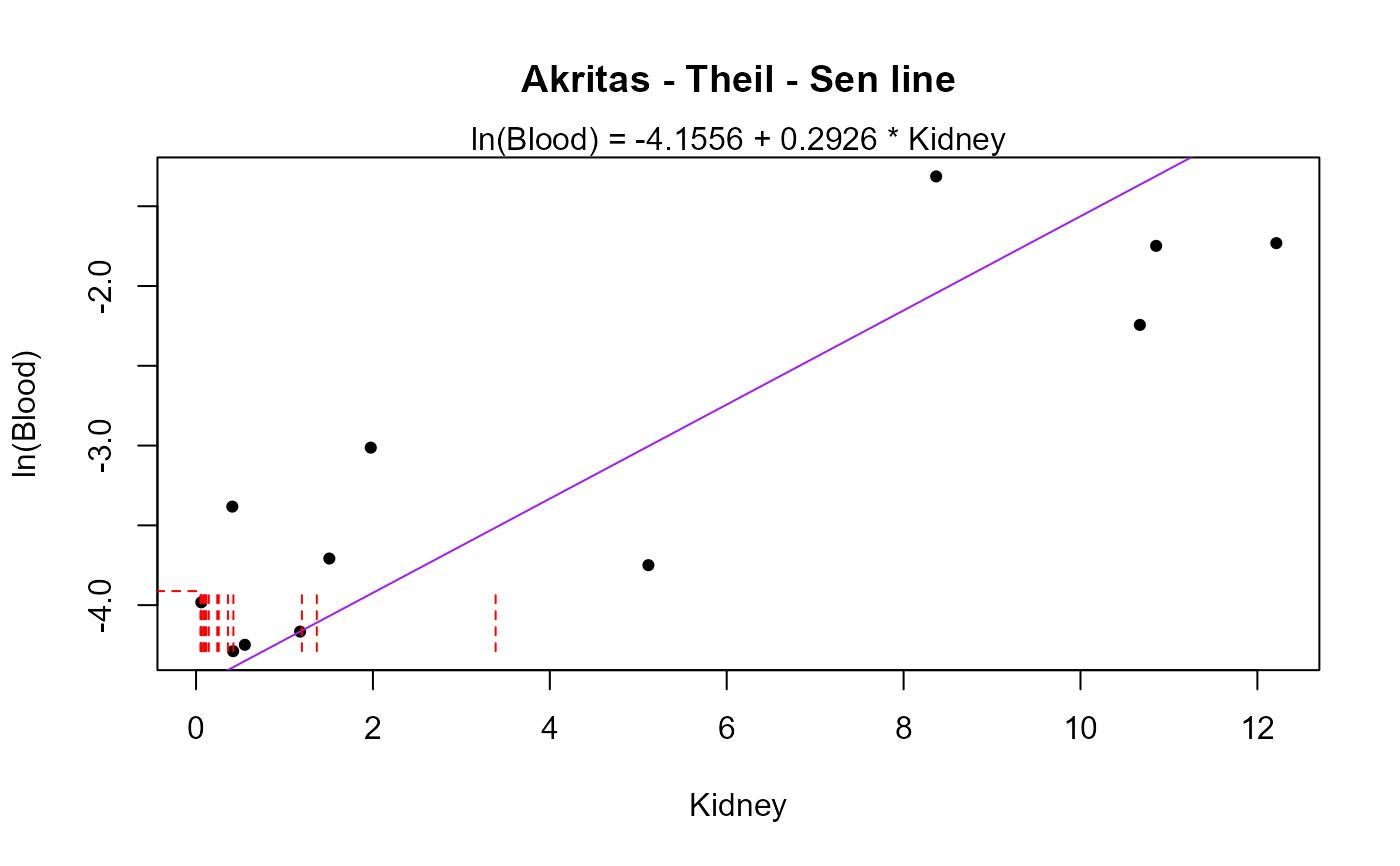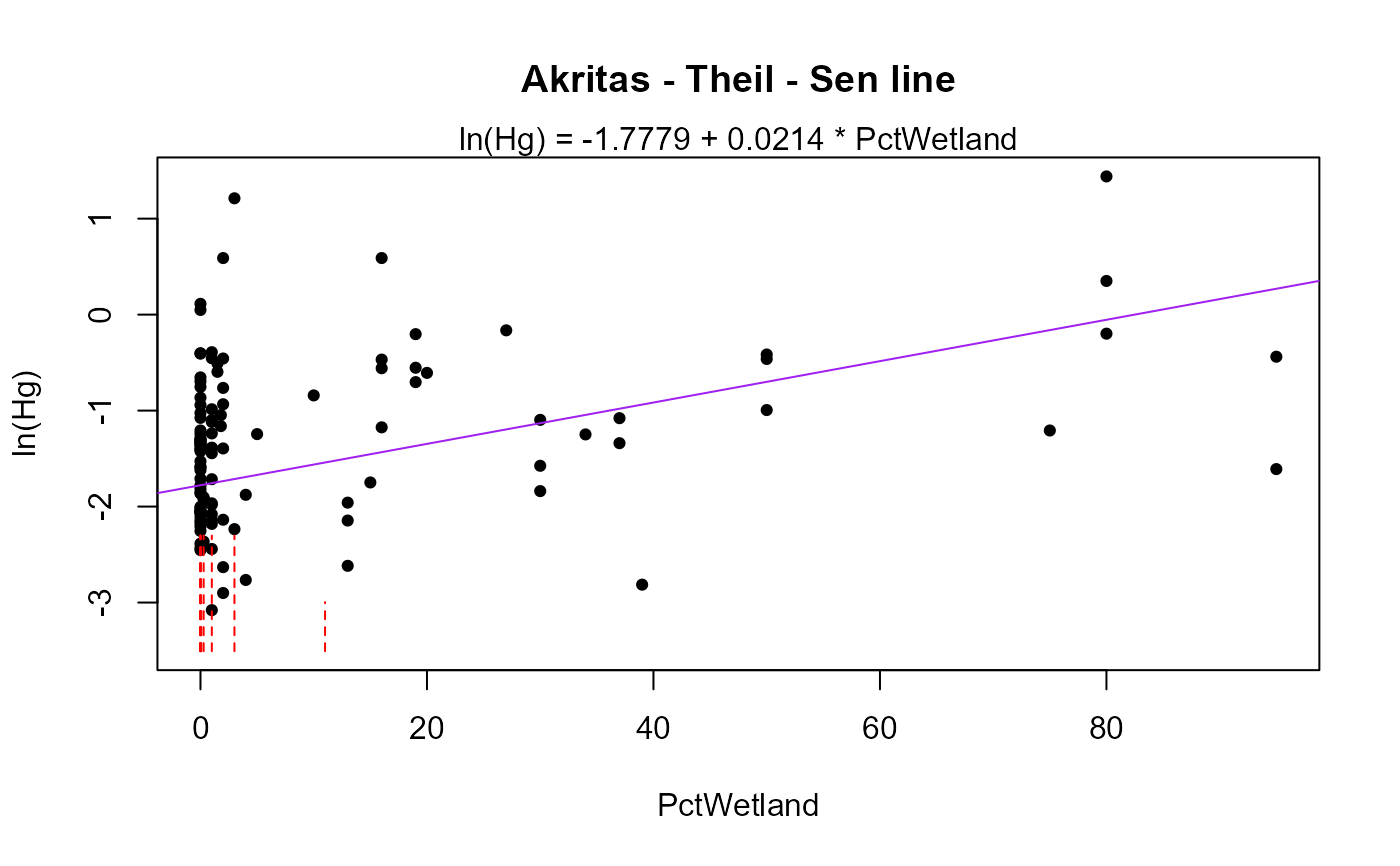Computes Kendall's tau and the Akritas-Theil-Sen (ATS) line for censored data, along with the test that the slope (and Kendall's tau) equal zero. For one x variable regression.

ATS(
y.var,
y.cen,
x.var,
x.cen = rep(0, times = length(x.var)),
LOG = TRUE,
retrans = FALSE,
xlabel = NULL,
ylabel = NULL,
printstat = TRUE
)

## Arguments

y.var

The column of y (response variable) values plus detection limits

y.cen

The y-variable indicators, where 1 (or TRUE) indicates a detection limit in the y.var column, and 0 (or FALSE) indicates a detected value in y.var.

x.var

The column of x (explanatory variable) values plus detection limits

x.cen

The x-variable indicators, where 1 (or TRUE) indicates a detection limit in the x.var column, and 0 (or FALSE) indicates a detected value in x.var.

LOG

Indicator of whether to compute the ATS line in the original y units, or for their logarithms. The default is to use the logarithms (LOG = TRUE). To compute in original units, specify the option LOG = FALSE (or LOG = 0).

retrans

Indicator of whether to retransform the plot and line back to original Y-variable units. Not needed when LOG = FALSE. When retrans = FALSE & LOG = TRUE the plot is drawn with logY units (default). When retrans = TRUE & LOG = TRUE the plot is drawn with original Y units.

xlabel

Custom label for the x axis of plots. Default is x variable column name.

ylabel

Custom label for the y axis of plots. Default is y variable column name.

printstat

Logical TRUE/FALSE option of whether to print the resulting statistics in the console window, or not. Default is TRUE.

## Value

Coefficients (intercept and slope) for the ATS line are printed, along with Kendall's tau correlation coefficient, test statistic S, and the (single) p-value for the test that tau and slope both equal zero. A scatterplot with the fitted trend-line superimposed is also drawn.

## Examples

# Both y and x are censored
data(PbHeron)
with(PbHeron, ATS(Blood, BloodCen, Kidney, KidneyCen))
#> Akritas-Theil-Sen line for censored data
#>
#> ln(Blood) = -4.1556 + 0.2926 * Kidney
#> Kendall's tau = 0.4217   p-value = 0.00043
#># x is not censored
data(Brumbaugh)
with(Brumbaugh,ATS(Hg, HgCen, PctWetland))
#> Akritas-Theil-Sen line for censored data
#>
#> ln(Hg) = -1.7779 + 0.0214 * PctWetland
#> Kendall's tau = 0.192   p-value = 0.00063
#>